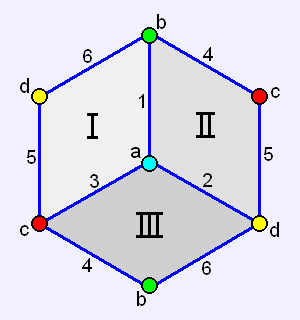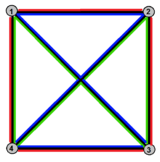# Hemicube (geometry)

Not to be confused with Demicube.
HemicubeType abstract regular polyhedron
globally projective polyhedron
Faces 3 squares
Edges 6
Vertices 4
Vertex configuration 4.4.4
Schläfli symbol {4,3}/2 or {4,3}3
Symmetry group S4, order 24
Dual polyhedron hemi-octahedron
Properties non-orientable
Euler characteristic 1

In abstract geometry, a hemicube is an abstract regular polyhedron, containing half the faces of a cube.

It can be realized as a projective polyhedron (a tessellation of the real projective plane by three quadrilaterals), which can be visualized by constructing the projective plane as a hemisphere where opposite points along the boundary are connected and dividing the hemisphere into three equal parts.

It has three square faces, six edges, and four vertices. It has an unexpected property that every face is in contact with every other face on two edges, and every face contains all the vertices, which gives an example of an abstract polytope whose faces are not determined by their vertex sets.

From the point of view of graph theory the skeleton is a tetrahedral graph, an embedding of K4 (the complete graph with four vertices) on a projective plane.

The hemicube should not be confused with the demicube – the hemicube is a projective polyhedron, while the demicube is an ordinary polyhedron (in Euclidean space). While they both have half the vertices of a cube, the hemicube is a quotient of the cube, while the vertices of the demicube are a subset of the vertices of the cube.

## Related polytopes

The hemicube is the Petrie dual to the regular tetrahedron, with the four vertices, six edges of the tetrahedron, and three Petrie polygon quadrilateral faces. The faces can be seen as red, green, and blue edge colorings in the tetrahedral graph: